Subsections

# The Definite Integral

## Purpose

The purpose of this lab is to introduce you to the definite integral and to Maple commands for computing definite integrals.

## Background

### Introduction

There are two main ways to think of the definite integral. The easiest one to understand, and the one we'll consider first, is as a means for computing areas (and volumes). The second way the definite integral is used is as a sum. That is, we use the definite integral to add things up''. Here are some examples.
• Computing net or total distance traveled by a moving object.
• Computing average values, e.g. centroids and centers of mass, moments of inertia, and averages of probability distributions.

Of course, when we use a definite integral to compute an area or a volume, we are adding up area or volume. So you might ask why make a distinction? The answer is that the notion of an integral as a means of computing an area or volume is much more concrete and is easier to understand.

You have learned in class that the definite integral is actually defined as a (complicated) limit of sums, so it makes sense that the integral should be thought of as a sum. You have also learned in class that the indefinite integral, or anti-derivative, can be used to evaluate definite integrals. Students often concentrate on techniques for evaluating integrals, and ingnore the definition of the integral as a sum. This is a mistake, for the following reasons.

1.
Many functions don't have anti-deriviatives that can be written down as formulas. Definite integrals of such function must be done using numerical techniques, which always depend on the definition of the integral as a sum.
2.
In many applications of the integral in engineering and science, you aren't given the function which is to be integrated and must derive it. The derivation always depends on the definition of the integral as a sum. You will see examples of this later on in the course.

### Definite and indefinite integrals with Maple

The basic Maple command for computing definite and indefinite integrals is the int command. The syntax is very similar to that of the leftsum and rightsum commands, except you don't need to specify the number of subintervals. Suppose you wanted to compute the following definite integral with Maple.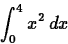The command to use is shown below.

  > int(x^2,x=0..4);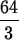Notice that Maple gives an exact answer, as a fraction. If you want a decimal approximation to an integral, you just put an evalf command around the int command, as shown below.
  > evalf(int(x^2,x=0..4));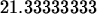To compute an indefinite integral with Maple, you just leave out the range for the limits of integration, as shown below.

  > int(x^2,x);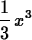Note that Maple does not include a constant of integration.

You can also use the Maple int command with functions or expressions you have defined in Maple. For example, suppose you wanted to find area under the curve of the function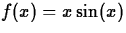on the interval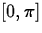. Then you can define this function in Maple with the command

  > f := x -> x*sin(x);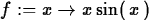and then use this definition as shown below.
  > int(f(x),x=0..Pi);You can also simply give the expression corresponding to f(x) a label in Maple, and then use that label in subsequent commands as shown below. However, notice the difference between the two methods. You are urged you to choose one or the other, so you don't mix the syntax up.

  > p := x*sin(x);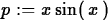> int(p,x=0..Pi);### More on computing definite integrals with Maple

The same int command works to compute any definite integral, whether it corresponds to an area or not. For example, to compute the definite integralyou could use the following Maple command.
  > int((2*x-3)^5,x=-2..4);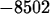Sometimes you need to compute a definite integral involving a piecewise-defined function. For example, suppose you have a function f(x) defined as followsand you needed to compute the definite integralThe best way to do this in Maple is to split it up into two integrals and use the appropriate formula, as shown below. How you split the integral up is determined by where the formula defining the function changes.
  > int(2-x^2,x=-5..1)+int(x,x=1..5);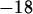### Definite integrals and average values

If a function f is integrable over an interval [a,b], then we define the average value of f, which we'll denote as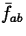,on this interval to be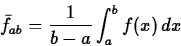Note that the average value is just a number. Note furthermore that we can rearrange the definition to give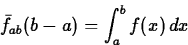If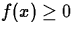on [a,b], then the average value has the following geometrical interpretation:is the height of a rectangle of width b-a such that the area of this rectangle is equal to the area under the graph of f from a to b. The following example shows you how to compute an average. The last plot command shows the function and the top of this rectangle.

  > f :=x ->  x*sin(x) ;> plot(f(x),x=0..Pi);

  > f_ave := int(f(x),x=0..Pi)/Pi;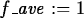> plot({f(x),f_ave},x=0..Pi);


## Exercises

1.
Use Maple to compute the following definite integrals.
(a)(b)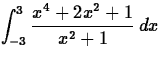Explain why your answer to this part is exactly twice your answer to the first part of this exercise.
2.
It is easy to see that if f(x)=0, thenHowever, it is not true that having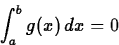implies g(x) is identically zero on the interval [a,b]. Explain why this is so, and come up with a function and an interval of your own that illustrates this. That is, find a function g(x), and an interval [a,b] such thatbut it is not true that g(x) is identically zero on the interval [a,b]. Include the integral and a plot of your function in your worksheet.

3.
Use Maple to find the area of the region bounded above by the curve y=x+2 and below by the curve y=x2-3x-3. Include a plot of the region.

4.
Consider the functionCompute the following integral.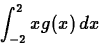5.
Suppose that the velocity of a particle moving in one dimension is given by

v(t) = -32 t + 15

Find the average velocity of the particle on the interval.Next: About this document ... Up: Labs and Projects for Previous: Labs and Projects for

Jane E Bouchard
3/31/2000